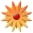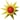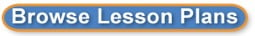# Linear Relationships in the Real World

Page Views: 407

Email This Lesson Plan to Me
Overall Rating:(5.0 stars, 1 ratings)

 Keywords: Math, Algebra 1 Subject(s): Grades 9 through 12 NETS-S Standard: Creativity and InnovationCommunication and CollaborationResearch and Information FluencyCritical Thinking, Problem Solving, and Decision MakingView Full Text of Standards School: Madison High School, Vienna, VA Planned By: Alyson Willey Original Author: Alyson Willey, Vienna
Subject: Patterns, Functions, and Algebra
SOL(s):
8.15
The student will:
a) Solve multi-step linear equations in one variable with the variable on one and two sides of the equation;
b) Solve two-step linear inequalities and graph results on a number line; and
c) Identify properties of operations used to solve an equation.

Objective:
The student will use problem solving, mathematical communication, mathematical reasoning, connections and representations to solve multi-step equations in one variable with the variable on one of two sides of the equations while identifying at least 3 careers which utilize this skill.

Materials:
SMARTboard, �Why do we need to know Linear Equations?� and �Linear Equations� graphic organizers, �Linear Equations in the real-world� worksheet, Exit ticket.

Opening Statement:
Why is it important for us to learn how to solve linear equations? What is a linear equation? These are questions you, as students, may be asking yourselves, thinking, �I will never use this later in my life.� Today, we will learn what a linear equation is and how you will use important skills, like problem-solving and making predictions, learned in the future, as well as what kind of careers incorporate the use linear equations to help them in their everyday jobs.

Lesson Body: 1-90 minute class period
Step Description Evidence-Based Strategy Time
1 -SMARTboard presentation correlating with graphic organizer, �Why do we need to know Linear Equations?� (examples: problem-solving skills, prediction skills) Direct instruction/ Whole group instruction 10 minutes
2 -In groups of 3-4, and on poster paper, make a list of possible careers where you would need to know problem-solving and predictions skills.
-Teacher provides examples such as Engineers, Bankers, and Architects
-Students share with the class Cooperative Learning 20 minutes
3 -SMARTboard instruction: �Linear Equations�
-Examples of solving multi-step linear equations are shown
- Video: �How to Solve Linear Equations in Algebra�
http://www.dummies.com/how-to/content/how-to-solve-simple-linear-equations-in-algebra.html
Direct
Instruction

Graphic Organizers 25 minutes
4 -In partners students complete �Linear Equations in the real-world� worksheet, solving 4 real-life, career-related problems in solving multi-step, linear equations (examples: calculating the dimensions of building a bridge and paying a taxi driver)
-Teacher monitors progress and goes over correct answers Think-Pair-Share

Scaffolding

Guided Practice 25 minutes
5 -Students start on homework #1, �Solving multi-step linear equations.�
-Teacher monitors progress
-Exit ticket: �Name one thing you learned from this lesson and two-related careers� and �What did you like about this lesson?� Independent Practice

Scaffolding 10 minutes

Students with Learning Disabilities (LD) and Emotional Disabilities (ED) will be given certain accommodations as deemed on their Individual Education Plan (IEP). Modifications involving assignments will be used as well. The following adaptations and modifications will be utilized in class:
� The use of graphic organizers and a copy of the teacher�s notes will be given to students who require it.
� Students will be divided into leveled groups during cooperative learning so that students who are struggling with the material may be paired with those who are stronger in the subject matter.
� Students will be given specific jobs or roles within group assignments so that there is a clear outline of expectations and limits.
� Homework will be modified for students who need it.

Closing:
Today we have explored the subject of multi-step linear equations and how they are applied in the real world. There are many jobs, which utilize the problem solving, and prediction skills necessary in learning linear equations. For homework, you will find more real life examples of solving linear equations and careers that incorporate them. Look back at your notes for help if you need it.

Student Assessment:
The students will be assessed based on the measurable objective, �The student will use problem solving, mathematical communication, mathematical reasoning, connections and representations to solve multi-step equations in one variable with the variable on one of two sides of the equations while identifying at least 3 careers which utilize this skill.� Students will be assessed through participation and observation in partner and group work. The teacher will collect group work, looking to see that at least 3 careers were identified. They will be given informal assessment grades for the �Linear equations in the real world worksheet� and the homework assignment given at the end of the day. Evaluation of the homework and group work will allow the teacher to decide what aspects of the lesson to re-visit.

Teacher Evaluation:
The teacher will utilize various evidence-based practices and differentiated instruction to meet the needs of the students in the classroom. Students will be given clear expectations and outlines for in-class and homework assignments. The teacher will determine understanding through observation and scaffolding during group work and the homework given. The exit ticket will be used as a way of determining the student�s interest of the lesson and understanding of related careers.

Follow-up Activities:
Students will complete a warm-up at the beginning of class with three examples of multi-step equations, adding 3 careers that use linear equations in their jobs and how. The teacher will ask for volunteers to relate the equations to possible careers. The warm-ups will be collected and assessed for understanding. The teacher will go over the homework on the SMARTboard to determine understanding, asking students to identify which problems they did not understand.
 Comments This program would help promote the concept of highly engaged learners with a student special education population that struggles in the area of math. With the addition of the Easiteach Next Generation Software, students will be able to explore real world concepts involving math which is so essential to their overall understanding and retention. Currently, students in special education math are some of the most underachieving sub groups involving standardized testing and overall math retention. The addition of this software program would help build problem solving and life skills for our special education math population. Cross-Curriculum Ideas Follow up activities regarding careers across content areas such as English, History and Science. In English, write about the student's choice of career in a multi-paragraph format. In History, research the origin of this career and why it is relevant to the real world. In Science, how math and science relate and have an impact on one another. Follow-Up Lesson: Solve two-to-four step linear equations in on variable using concrete materials Career-related activity: calculate cost, revenue and margin of profit for two different businesses (Banker) Lesson: Solve two-step inequalities in one variable Job-related group work: Architect-Use information solved to find the correct scale of a building and draw a blueprint Materials: Whiteboards, Graphing, High Other Items: 1 Easiteach Next Generation (PC)- Interactive Presentation Software for Whiteboards and more, \$179.95 each, total of \$179.95